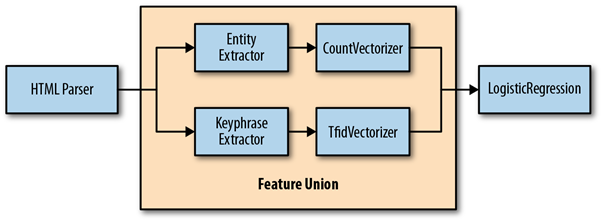# Predictors and FeatureUnion in ML

Scikit-learn refers to machine learning algorithms as estimators. There are three different types of estimators: classifiers, regressors, and transformers. The inheritance of the second class determines what kind of estimator the model represents. We’ll divide the estimators into two groups based on their interface. These two groups are predictors and transformers, and in this blog, we’ll discuss Predictors.

## Predictors: classifiers and regressors

As the name suggests, predictors are models that make predictions. There are two main methods: Fit (X, y): trains/fit the object to the feature matrix X and label vector y and Predict (X): makes predictions on the passed data set X.

Code :

```				```
from sklearn.linear_model import LinearRegression

# create model and train/fit
model = LinearRegression()
model.fit(X, y)

# predict label values on X
y_pred = model.predict(X)
print(y_pred)
print("shape of the  prediction array: {}".format(y_pred.shape))
print("shape of the training set: {}".format(X.shape))
```
```

There is one thing to note that the output of predict(X) is a NumPy array of one dimension.

## Pipelines

Before discussing feature unions, let’s first have a brief knowledge about Pipelines. As our analysis and workflow become more complicated, we need a tool that helps with scaling up. For example, suppose you need to apply multiple transformations to your data before using a supervised machine learning model. In that case, you can apply the transformations explicitly, creating intermediate variables of the transformed data. But this work is a little bit tiresome.

Here, the Pipelines approach helps prevent keeping track of intermediate transformations and scales up our code for more complicated analysis. Essentially, a pipeline is an estimator object that holds a series of transformers with a final estimator.

Code Example:

```				```
from sklearn.pipeline import Pipeline
from sklearn.preprocessing import PolynomialFeatures

# construct pipeline
scaler = StandardScaler()
poly_features = PolynomialFeatures(degree=2)
lin_reg = LinearRegression()

pipe = Pipeline([
('scaler', scaler),
('poly', poly_features),
('regressor', lin_reg)
])
```
```

## Feature Union

A FeatureUnion is another tool for dealing with situations where your data requires different transformation processes for various features. Because in ColumnTransformer, processes feature separately and combine the results into a single feature matrix, but in feature union, it can handle more complex workflows where you need to use distinct transformers and estimators together before passing the complete feature matrix to a final estimator.We already know that Pipeline objects arrange estimators in a series, but FeatureUnion objects arrange transformers in parallel. A FeatureUnion object combines the output of each of the transformers in parallel to generate one output matrix. Using a combination of Pipeline and FeatureUnion objects, we can construct complicated machine learning workflows within a single scikit-learn estimator object. So, the ultimate aim of feature union is to combine several feature extraction mechanisms into a single transformer.

```				```
from sklearn.pipeline import FeatureUnion
from sklearn.decomposition import PCA, TruncatedSVD

union = FeatureUnion([("pca", PCA(n_components=1)),
("svd", TruncatedSVD(n_components=2))])

X = [[0., 1., 3], [2., 2., 5]]
union.fit_transform(X)
array([[ 1.5   ,  3.0...,  0.8...],
[-1.5    ,  5.7..., -0.4...]])
```
```### Get free consultation from our tech experts## Related articles you may would like to read

##### Will Driving Automation Become a Vital Part of The Future?##### Autonomous Car in Automotive### FREE eBOOK: Recommended RFP process for selecting cloud provider

We are here to simply your migration to cloud by embarking on a systematic, planned, stage-wise journey to the cloud.

##### Categories